Electron. J. Diff. Eqns., Vol. 2007(2007), No. 48, pp. 1-19.

### Existence and asymptotic expansion of solutions to a nonlinear wave equation with a memory condition at the boundary Nguyen Thanh Long, Le Xuan Truong

Abstract:
We study the initial-boundary value problem for the nonlinear wave equation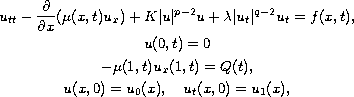where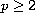,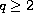,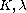are given constants and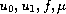are given functions. The unknown function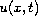and the unknown boundary value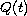satisfy the linear integral equation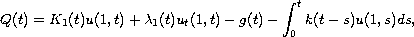where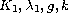are given functions satisfying some properties stated in the next section. This paper consists of two main sections. First, we prove the existence and uniqueness for the solutions in a suitable function space. Then, for the case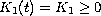, we find the asymptotic expansion in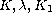of the solutions, up to order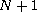.

Submitted October 27, 2006. Published March 20, 2007.
Math Subject Classifications: 35L20, 35L70.
Key Words: Nonlinear wave equation; linear integral equation; existence and uniqueness; asymptotic expansion.

Show me the PDF file (308K), TEX file, and other files for this article.Nguyen Thanh Long Department of Mathematics Hochiminh City National University 227 Nguyen Van Cu, Q5, HoChiMinh City, Vietnam email: longnt@hcmc.netnam.vn, longnt2@gmail.com Le Xuan Truong Department of Mathematics, Faculty of General Science University of Technical Education in HoChiMinh City 01 Vo Van Ngan Str., Thu Duc Dist., HoChiMinh City, Vietnam email: lxuantruong@gmail.com